# NXT Color Sorter Program

## Introduction

The NXT 2.0 color sorter model provides a good example for simple, state machine, software design. The color sorter sorts the different color balls each into their own tray by color as shown in the following video.

The following discussion describes the state machine approach and resulting NXT-G program. The NXT-G program may be downloaded at the end of this article.

## Developing a State Table

The following diagram schematically represents the sorter tray that catches the balls from the dispenser tray. The arrow represents the chute down which the ball rolls into the sorter tray. The number represents the color ball that each compartment of the sorter tray catches: compartment 2 catches the blue ball, compartment 3 catches the green ball, compartment 4 catches the yellow ball, and compartment 5 catches the red ball. Note that the compartment numbers match the number of each color of ball as returned by the color sensor (see table 1).

Table 1
ColorCode
Blue2
Green3
Yellow4
Red5

The first step is to determine what the tray must do given its current position, and the ball that is "on deck" to roll down the chute. For example, the tray is currently positioned with compartment 2 (blue) under the chute and the yellow ball (number 4) is on deck in the chute. The tray must make one half (0.50) rotation in the counter-clockwise (or clockwise) direction so that compartment 4 will be correctly positioned to catch the yellow ball. Note that counter-clockwise directions are assigned negative values. This scheme is optimal in terms of minimizing the amount of rotation that the tray must do to catch in the correct compartment the ball currently on deck.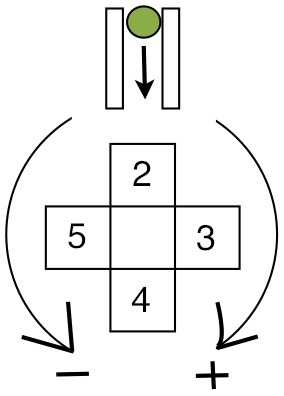Diagram 1. Numbering scheme for the four compartments
in the color sorter tray. Each tray gets balls of the same color.

Table 2 summarizes all sixteen possible combinations of sorter tray compartments and balls on deck in the chute. Table 2 represents an actual state table that could be implemented with software. "Current state" is identically the number of the compartment currently under the chute, "Ball Dispensed" is the ball on deck ready to roll down the chute, and "Rotation of Ball Catcher Tray" is the amount of rotation required to bring the correct sorter tray compartment into position to catch the ball. For example, if the Current State is 3, meaning that the sorter tray compartment for green balls is currently positioned under the chute, and the yellow ball is on deck, then the sorter tray must move 0.25 rotation in the counter-clockwise direction, as indicated by the minus sign.

Table 2
Current State Ball Dispensed Rotation of Ball
Catcher Tray
Ball Dispensed -
Current State + 3
2203
23-0.254
240.55
250.256
320.252
3303
34-0.254
350.55
420.51
430.252
4403
45-0.254
52-0.250
530.51
540.252
5403

Can table 2 be simplified? One way to approach this question involves looking for a formula that can mathematically map each combination to a specific action, namely a partial rotation of the tray. Consider the formula:

x = ball dispensed - current state + 3

where Ball Dispensed is the ball on deck in the chute, and Current State is the compartment of the sorter tray under the chute. Note the numbers defined by this formula each correspond to a unique rotation of the tray. For example, 2 always corresponds to a 0.25 rotation of the tray in every case. In fact, the formula above defines a function mapping the integers 0 through 6 to the set containing the rotations -0.25, 0, 0.25, 0.5. That is

f({x}) = f{0,...,6} → { -0.25, 0, 0.25, 0.5 }

This mapping provides a much simplified state table that can be more easily implemented in software. Table 3 below is exactly the simplified state table.

Table 3
Ball Dispensed -
Current State + 3
Rotation of Sorter
Tray
0-0.25
10.5
20.25
30
4-0.25
50.5
60.25

## NXT-G Program

Figure 1 shows the first half of the NXT-G program implementing the state table color sorting algorithm. The blocks from the start to the color sensor have the main function of aligning the color sorter tray and the color ball dispenser at a known physical position. After the sorter tray and the chute are aligned, the chute is tipped forward to allow a colored ball to roll into the chute. The value of the color sensor gets stored in the variable "BallColor". The value in BallColor then gets tested by a range block to make sure that color sensor has, indeed, sensed one of the color balls. If a ball does not roll into the chute, and the chute is empty, the color sensor will return a value of 0. In this case the sorter tray will not be rotated, as can be seen in figure 2, where the input to the IF block is the output of the range block in figure 1.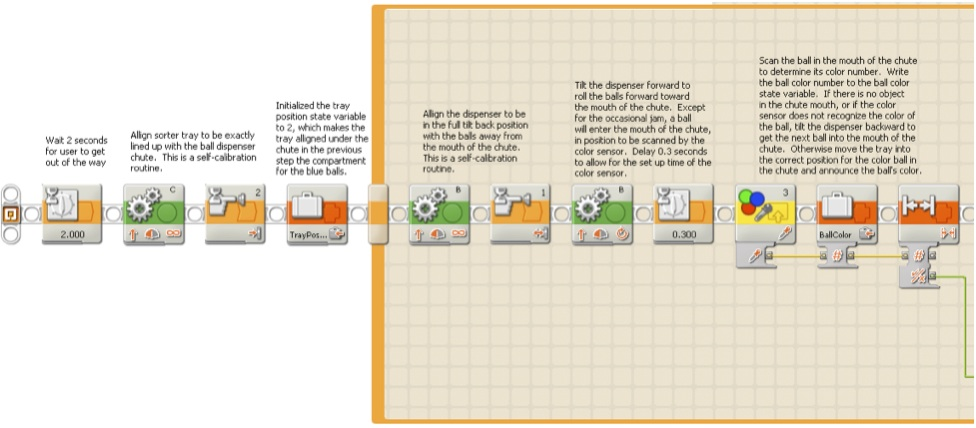Figure 1.

Figure 2 shows the second half of the NXT-G program. There are two case statement structures in the second half of the program. The value of BallColor selects one of four cases according to the value returned previously by the color sensor. Each case plays a sound which gives an audible indicate of the color of the ball currently on deck. Following the section of code which annunciates the ball color, are three blocks which calculate the value of the formula discussed above. The first block reads the value of CurrentState, the next block adds this value to the value stored in the BallColor variable, and finally the third block adds 3. The result of this calculation is used to select one of seven cases. Each case has a motor block set to rotate the motor shaft the amount shown in table 3 (see figure 3). Note that case three involves zero rotation, essential a "do nothing" case. A display block has been inserted in place of a motor block in this case.

Lastly, the value of BallColor variable gets written to CurrentState variable. The case structure above has rotated the sorter tray compartment so that the compartment number under the dispenser chute is the same as BallColor. Hence the new current state is identically equal to BallColor.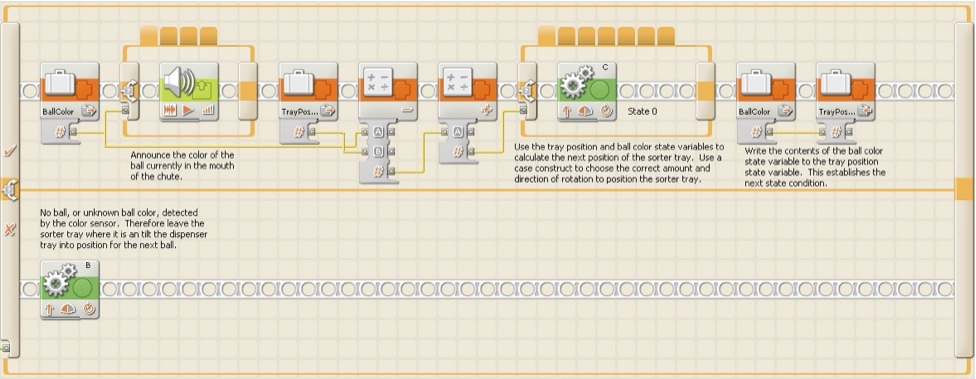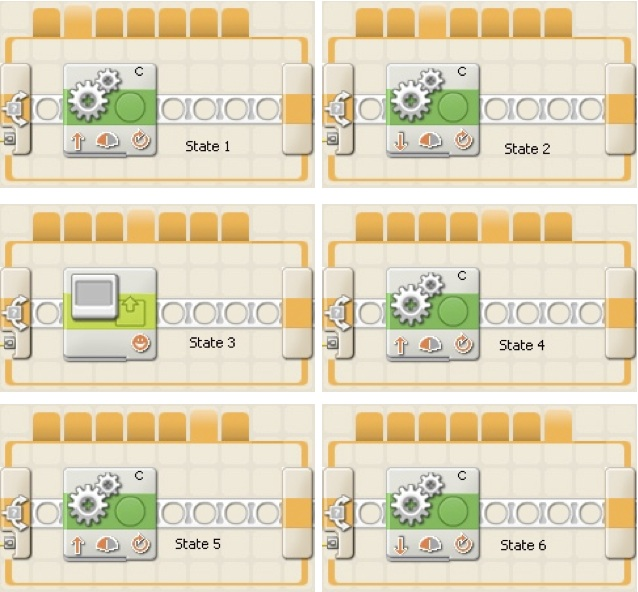Figure 2

## The Challenge

Using the state machine concepts discussed above, write a NXT-G program that dispenses four balls, each a different color, to each one of three color sorter tray compartments. For example compartment 2 has one blue, one green, one yellow, and one red ball; compartment 3 similarly has one blue, one green, one yellow, and one red ball; and etc. The end result should be similar to that shown in figure 4.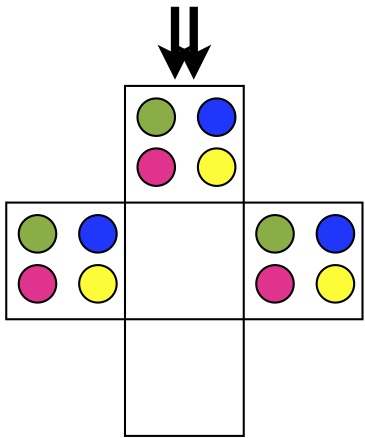Figure 4. Can you write a NXT-G program that
arranges the color balls as shown above?